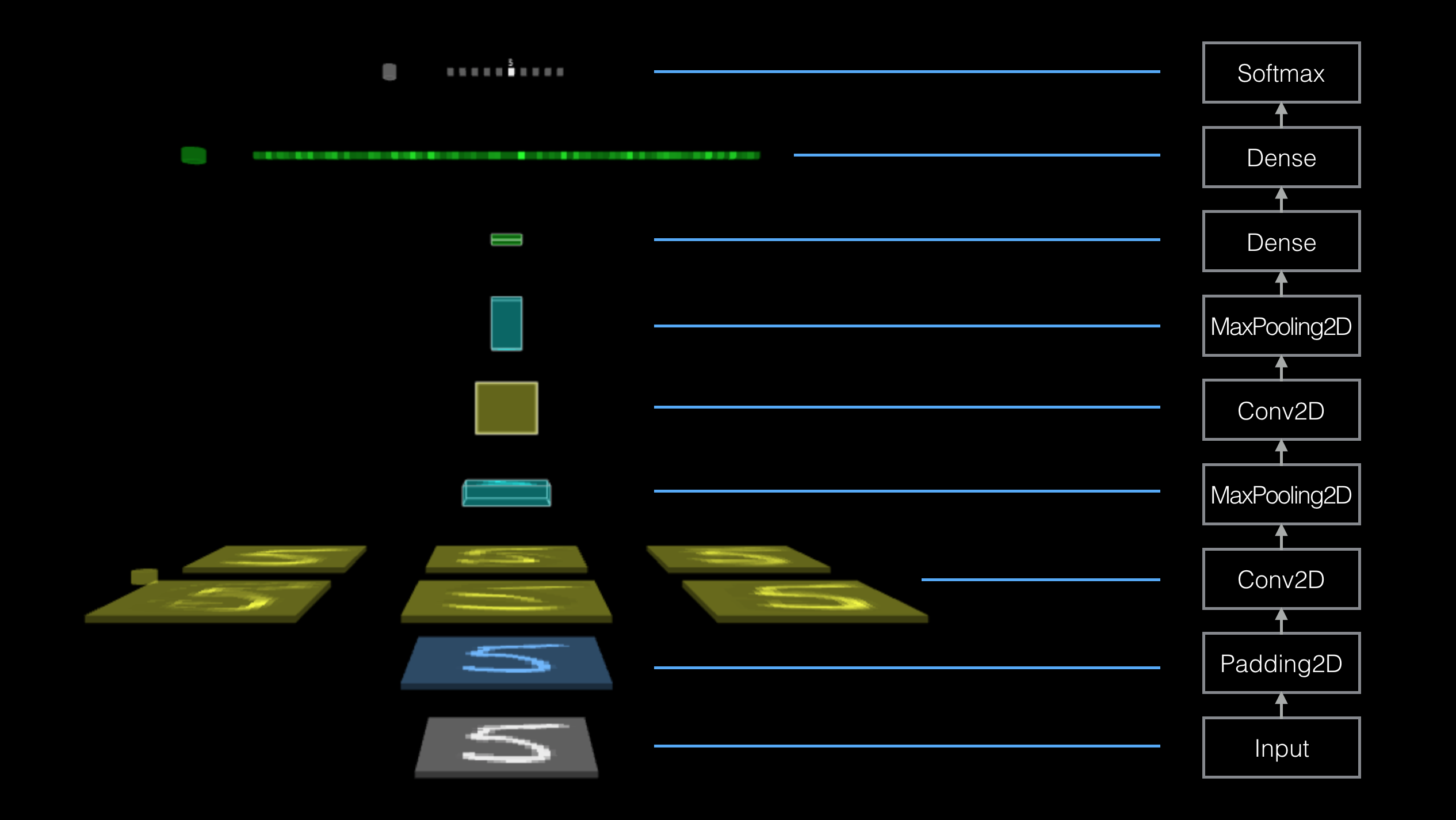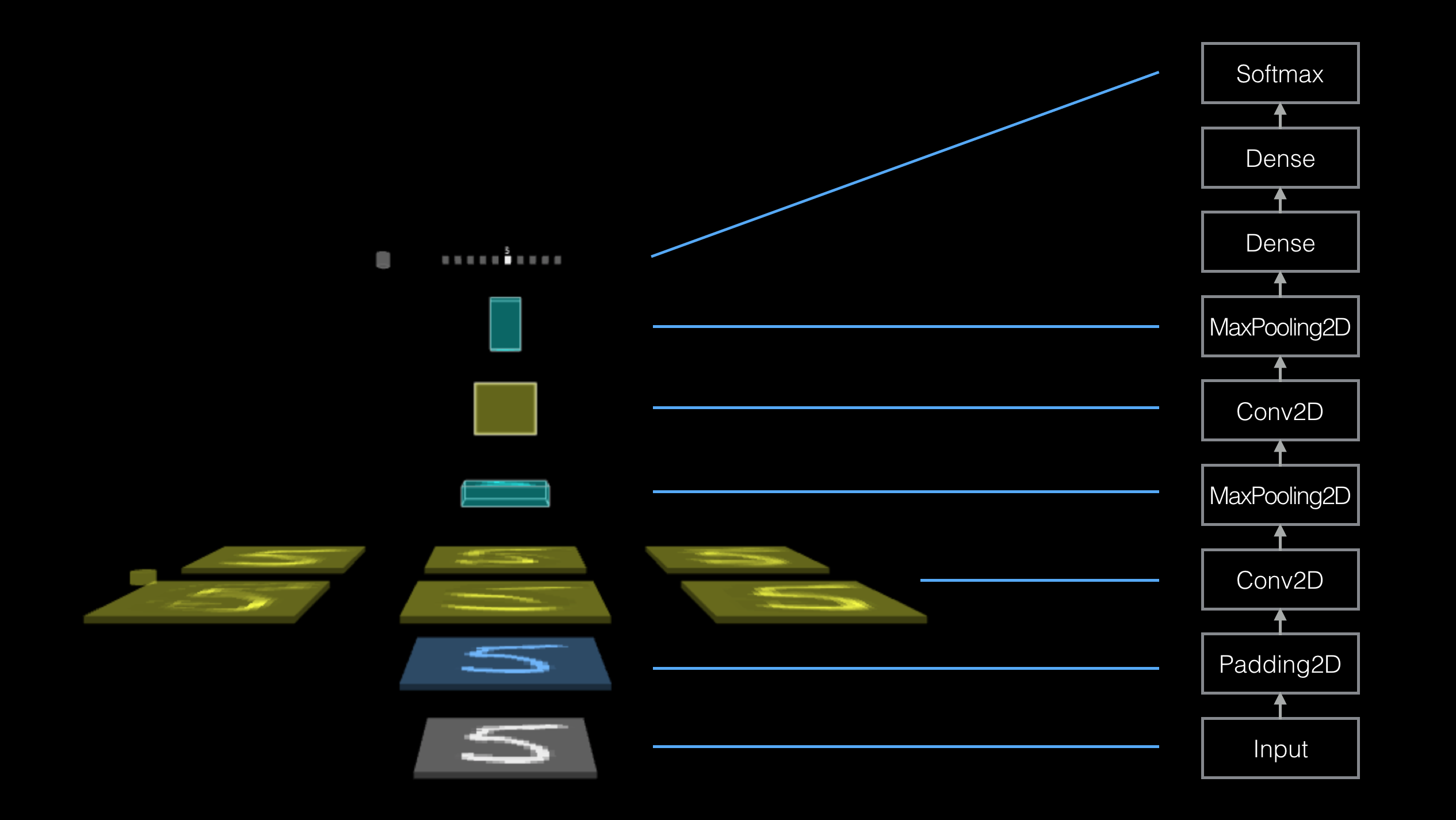TensorSpace.jsTensorSpace Converter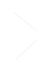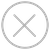TensorSpace 顺序模型和 Keras 中的顺序模型有着相似的概念 -- 多个网络层的线性堆叠。在 TensorSpace 中，我们可以使用顺序模型的 .add() 方法来将 TensorSpace Layer 添加到模型中，TensorSpace 将会在初始化阶段自动构建出 Layer 栈。``````let model = new TSP.models.Sequential( modelContainer );

outputs: [ "0", "1", "2", "3", "4", "5", "6", "7", "8", "9" ]
} ) );

});

model.init();``````

TensorSpace 函数模型和 Keras 中的函数模型有着相似的概念 -- 可用于定义复杂模型。在 TensorSpace 中，我们可以使用 TensorSpace Layer 的 .apply() API 和 网络层融合方法 来连接 Layer，然后配置模型的 inputsoutputs 参数来定义模型的输入层和输出层。TensorSpace 将会根据以上信息，在初始化阶段构建出可视化模型。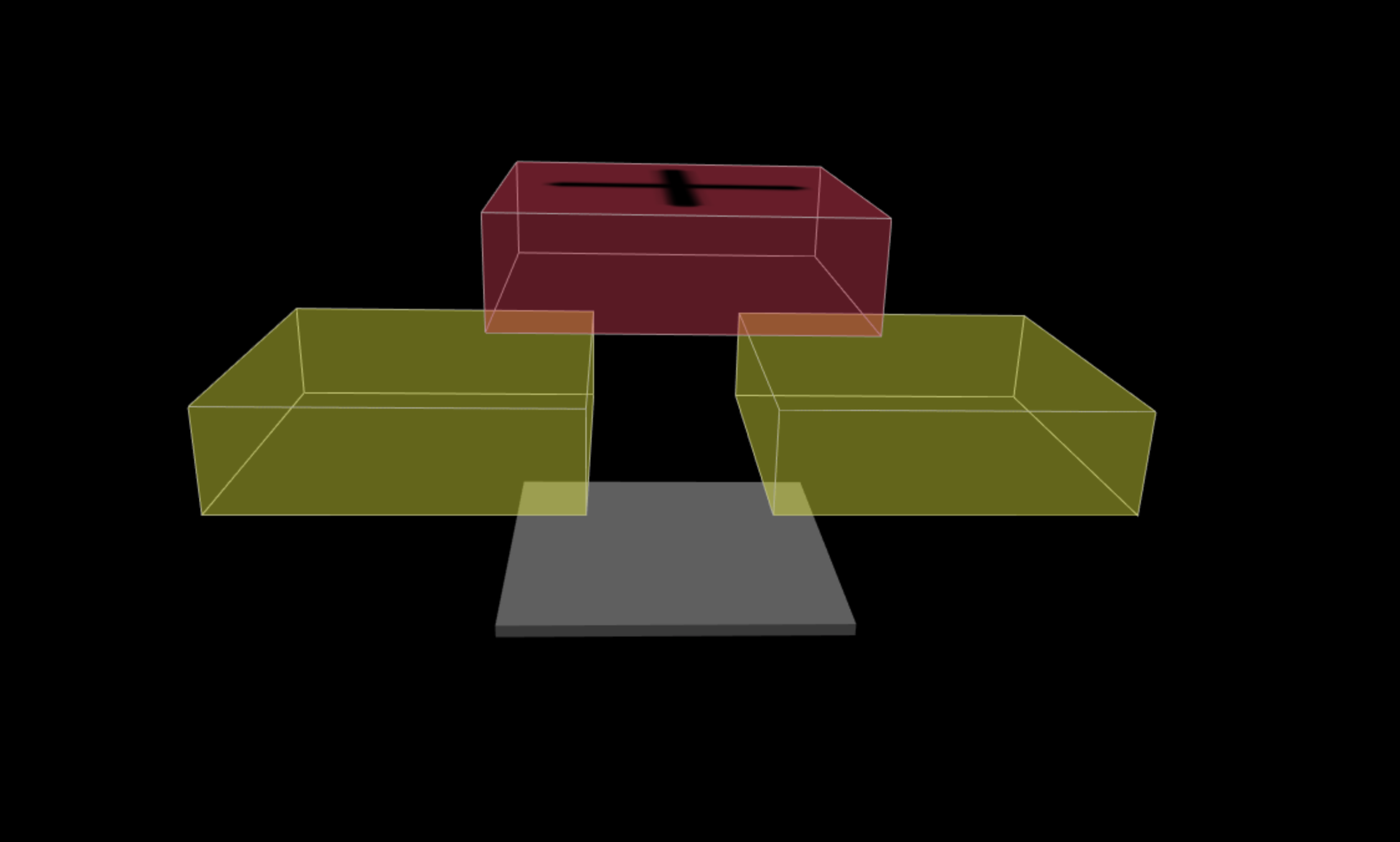``````let input = new TSP.layers.GreyscaleInput();

let conv2d_1 =  new TSP.layers.Conv2d();
conv2d_1.apply(input);

let conv2d_2 =  new TSP.layers.Conv2d();
conv2d_2.apply(input);

let model = new TSP.models.Model(modelContainer, {
inputs: [input],
});